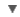POS
WORDNET DICTIONARY
CIDE DICTIONARY
OXFORD DICTIONARY
Link, Gadget and Share#ohm

RELATED WORDS :

:
Noun

## CIDE DICTIONARY

ohmn. [So called from the German electrician, G. S. Ohm.].
The standard unit in the measure of electrical resistance, being the resistance of a circuit in which a potential difference of one volt produces a current of one ampére. As defined by the International Electrical Congress in 1893, and by United States Statute, it is a resistance substantially equal to 109 units of resistance of the C. G. S. system of electro-magnetic units, and is represented by the resistance offered to an unvarying electric current by a column of mercury at the temperature of melting ice 14.4521 grams in mass, of a constant cross-sectional area, and of the length of 106.3 centimeters. As thus defined it is called the international ohm.  [1913 Webster]
Ohm's law (Elec.), the statement of the fact that the strength or intensity of an electrical current is directly proportional to the electro-motive force, and inversely proportional to the resistance of the circuit.

## OXFORD DICTIONARY

ohm, n. Electr. the SI unit of resistance, transmitting a current of one ampere when subjected to a potential difference of one volt.

Derivative
ohmage n.
Etymology
G. S. Ohm, Ger. physicist d. 1854
copyright © 2012 Yayasan Lembaga SABDA (YLSA) | To report a problem/suggestion